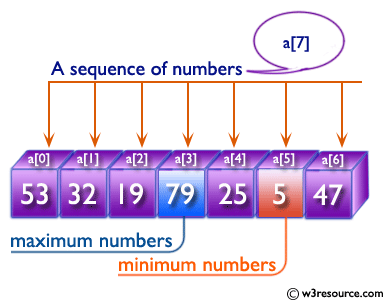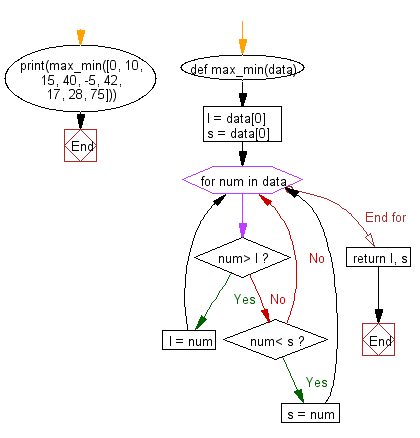﻿ Python: Find the maximum and minimum numbers from a sequence of numbers - w3resource# Python: Find the maximum and minimum numbers from a sequence of numbers

## Python Basic: Exercise-148 with Solution

Write a Python function to find the maximum and minimum numbers from a sequence of numbers.
Note: Do not use built-in functions.

Sample Solution :-

Python Code :

``````def max_min(data):
l = data
s = data
for num in data:
if num> l:
l = num
elif num< s:
s = num
return l, s

print(max_min([0, 10, 15, 40, -5, 42, 17, 28, 75]))
``````

Sample Output:

```(75, -5)
```

Pictorial Presentation:Flowchart:## Visualize Python code execution:

The following tool visualize what the computer is doing step-by-step as it executes the said program:

Python Code Editor :

Have another way to solve this solution? Contribute your code (and comments) through Disqus.

What is the difficulty level of this exercise?

Test your Python skills with w3resource's quiz

﻿

## Python: Tips of the Day

List comprehension:

```>>> m = [x ** 2 for x in range(5)]
>>> m
[0, 1, 4, 9, 16]
```